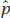# AP Statistics Multiple-Choice Practice Questions: Sampling Distributions 2

### Test Information9 questions21 minutes

1. Suppose using accelerometers in helmets, researchers determine that boys playing high school football absorb an average of 355 hits to the head with a standard deviation of 80 hits during a season (including both practices and games). What is the probability on a randomly selected team of 48 players that the average number of head hits per player is between 340 and 360?

2. It is estimated that school CO2 levels average 1200 ppm with a standard deviation of 300 ppm. In a random sample of 30 schools, what is the probability that the mean CO2 level is more than 1000, a level at which some researchers feel will cause a drop in academic performance?

3. Binge drinking is a serious problem, killing more than 1700 college students per year. Suppose the incidence of binge drinking is 43% and 37%, respectively, at two large universities. In a random sample of 75 students at the first school and 80 students at the second, what is the probability that the difference (first minus second) between the percentages of binge drinkers is between 5% and 10%?

4. Suppose "sleep-trained" babies (allowed to cry themselves to sleep) wake up an average of 1.2 times a night with a standard deviation of 0.3 times, while nontrained babies wake up an average of 1.8 times a night with a standard deviation of 0.5 times. In a random sample of 80 babies, half of which are sleep-trained, what is the probability that the nontrained babies in the sample wake up an average number of times greater than 0.75 more than the average of the sleep-trained babies?

5. Which of the following statements are true?

I.Like the normal, t-distributions are always symmetric.

II.Like the normal, t-distributions are always mound-shaped.

III.The t-distributions have less spread than the normal, that is, they have less probability in the tails and more in the center than the normal.

6. Which of the following statements about t-distributions are true?

I.The greater the number of degrees of freedom, the narrower the tails.

II.The smaller the number of degrees of freedom, the closer the curve is to the normal curve.

III.Thirty degrees of freedom gives the normal curve.

7. A study is to be performed to estimate the proportion of voters who believe the economy is "heading in the right direction." Which of the following pairs of sample size and population proportion p will result in the smallest variance for the sampling distribution of?

8. A random sample of 50 high school girls has a mean of 287 Facebook friends with a standard deviation of 68 friends. An independent random sample of 50 high school boys has a mean of 165 Facebook friends with a standard deviation of 45 friends. What is the standard error of the difference (girls - boys) between the sample means?

9. In a recent study of fatal automobile crashes ( for drivers ages 21-24, 32% were legally drunk, while for drivers ages 25-34, 29% were legally drunk. A simulation is conducted in which a random sample of 400 drivers ages 21-24 and a random sample of 400 drivers 25-34, all involved in fatal automobile crashes, are selected. The difference in proportions of those who were legally drunk is calculated, and this simulation is repeated 1000 times. Which of the following is most likely to occur in the simulated sampling distribution of the difference between the two sample proportions?